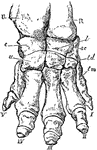### Elephant Foot

"Right fore foot of Indian Elephant. U, ulna; R, radius; c, cunelform; l, lunar; sc, scaphold; u, unciform;…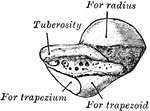### Left Scaphoid

A view of the left scaphoid seen from behind.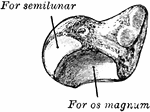### Left Scaphoid

A view of the left scaphoid seen from in front.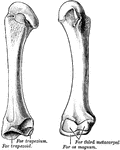### Metacarpal

The second metacarpal of the left hand.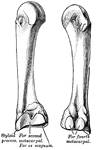### Metacarpal

The third metacarpal of the left hand.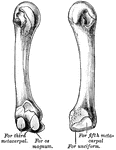### Metacarpal

The fourth metacarpal of the left hand.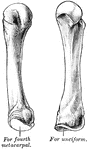### Metacarpal

The fifth metacarpal of the left hand.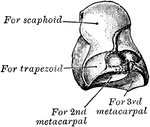### Os Magnum

The left os magnum, the outer side seen from below.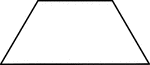### Large Trapezoid for Pattern Block Set

One large trapezoid for pattern block set.### Large Trapezoids for Pattern Block Set

Three large trapezoids for pattern block set.### Large Trapezoids for Pattern Block Set

Three large trapezoids for pattern block set.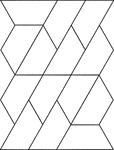### Small Trapezoids for Pattern Block Set

Fourteen small trapezoids for pattern block set.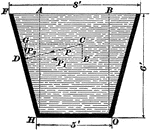### Oblique Surfaces

Pressure upon an oblique surface. In the image, pressure acts on all sides of the surface equally.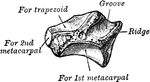### Trapezium

The left trapezium as seen from the front.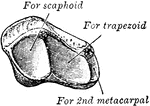### Trapezium

The left trapezium as seen from the above and the mesal side.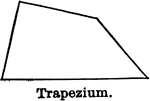### Trapezium

Illustration of a trapezium, a quadrilateral which has no two sides parallel.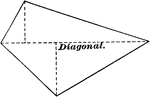### Trapezium

Illustration of a trapezium, a quadrilateral having no two sides parallel. The diagonal is labeled in…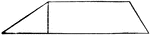### Trapezoid

A trapezoid is a quadrilateral having only two of its sides parallel.### Trapezoid

The left trapezoid as seen from the above, the inner side, and behind.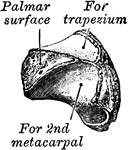### Trapezoid

The left trapezoid as seen from the front, below, and outer side.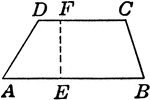### Trapezoid

Trapezoid with angles and height labeled.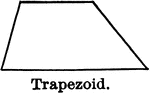### Trapezoid

Illustration of a trapezoid, a quadrilateral which has two and only two sides parallel.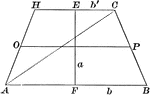### Area of a Trapezoid

Illustration of a trapezoid with altitude a and bases b and b' used to demonstrate that the area is…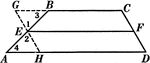### Line Bisecting Trapezoid Sides

Illustration used to prove the theorem "The line bisecting one of the non parallel sides of a trapezoid…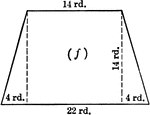### Trapezoid With Dimensions

Trapezoid with dimensions labeled. Trapezoid can be used to calculate area.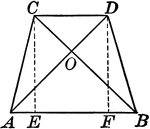### Isosceles Trapezoid With Equal Diagonals

Illustrations of an isosceles trapezoid with equal diagonals drawn.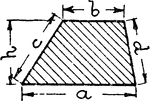### Trapezoid With Labels

An illustration of a trapezoid with 4 sides and height labeled.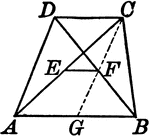### Trapezoid With Lines Joining the Midpoints of the Diagonals

Illustrations of a trapezoid with a line joining the midpoints of the diagonals.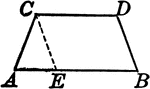### Trapezoid With Line Segment Parallel to One Side

Illustrations of a trapezoid with a line segment drawn parallel to one side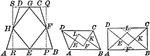### Trapezoids With Lines Joining Midpoints

Illustrations to show trapezoids with lines joining the midpoints of the sides of any quadrilateral,…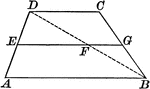### Trapezoid With Median

Illustration of a trapezoid, with the median drawn. The median of a trapezoid is parallel to the bases,…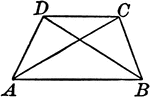### Trapezoid With Diagonals

Illustrations of a trapezoid with diagonals drawn.